# Expression of a variable from the formula + surface area - math problems

#### Number of problems found: 198

• Base side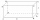In a quadrilateral prism, are known surface area S = 12400 mm2 base side m = 40mm and prism height = 120mm, what is the length of base side n =?
• The surfaceThe surface of the cylinder is 1570 cm2, its height is 15 cm. Find its volume and radius of the base.
• How toHow to find a total surface of a rectangular pyramid if each face is to be 8 dm high and the base is 10 dm by 6 dm.
• Frustrum - volume, areaCalculate the surface and volume of the truncated cone, the radius of the smaller figure is 4 cm, the height of the cone is 4 cm and the side of the truncated cone is 5 cm.
• Regular square prismThe volume of a regular square prism is 192 cm3. The size of its base edge and the body height is 1: 3. Calculate the surface of the prism.
• The surfaceThe surface of a truncated rotating cone with side s = 13 cm is S = 510π cm2. Find the radii of the bases when their difference in lengths is 10cm.
• The blockThe block, the edges formed by three consecutive GP members, has a surface area of 112 cm2. The sum of the edges that pass through one vertex is 14 cm. Calculate the volume of this block.
• Base diagonalIn a regular 4-sided pyramid, the side edge forms an angle of 55° with the base's diagonal. The length of the side edge is eight meters. Calculate the surface area and volume of the pyramid.
• Surface and volume - cubeFind the surface and volume of a cube whose wall diagonal is 5 cm long.
• The volumeThe volume of the cone is 94.2dm³, the radius of the base is 6 dm Calculate the surface of the cone.
• Truncated pyramidFind the volume and surface area of a regular quadrilateral truncated pyramid if base lengths a1 = 17 cm, a2 = 5 cm, height v = 8 cm.
• What is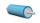What is the height of a cylinder whose surface size is 602.88 cm2 and the content of its shell is 376.8 cm2?
• The cylinder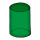In a rotating cylinder it is given: the surface of the shell (without bases) S = 96 cm2 and the volume V = 192 cm cubic. Calculate the radius and height of this cylinder.
• Rotary cylinderIn the rotary cylinder it is given: surface S = 96 cm2 and volume V = 192 cm cubic. Calculate its radius and height.
• Pentagonal pyramidThe height of a regular pentagonal pyramid is as long as the edge of the base, 20 cm. Calculate the volume and surface area of the pyramid.
• Truncated cone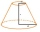Find the volume and surface area of the truncated cone if r1 = 12 cm, r2 = 5 cm and side s = 10 cm.
• Regular 4-sided pyramidFind the area (surface area) of a regular 4-sided pyramid if its height is 20 m and the wall height is 23 m.
• Cuboid - ratioFind the volume of a block whose dimensions are in the ratio 2: 3: 4 and the surface is 117 dm2.
• Prism height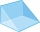What is the prism's height with the base of a right triangle of 6 cm and 9 cm? The diaphragm is 10.8 cm long. The volume of the prism is 58 cm3. Calculate its surface.
• Hard cone problemThe surface of the cone is 200 cm², its height is 7 centimeters. Calculate the volume of this cone.

Do you have an exciting math question or word problem that you can't solve? Ask a question or post a math problem, and we can try to solve it.

We will send a solution to your e-mail address. Solved examples are also published here. Please enter the e-mail correctly and check whether you don't have a full mailbox.

Expression of a variable from the formula - math problems. Examples for the calculation of the surface area of ​​the solid object .